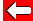# Sine (sin)

Now let's have a look at sin in use. Below is a right-angle triangle with a 30° angle marked and two sides. Recall "sohcahtoa"!
 By definition, sin 30°  sin 30° = opposite÷hypotenuse = 4÷8 = 0·5Sin 30° will always equal 0·5, when applied to right angle triangles. Now let's see how we use those pesky calculators...Go back a pageMaths MenuGo to next page